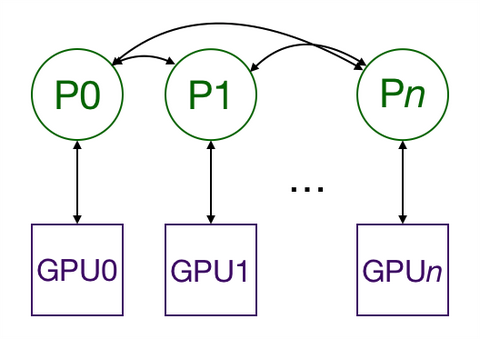## 薰风说

Distributed data parallel training in Pytorchyangkky.github.io## 2. Why Distributed Data Parallel？

Pytorch兼顾了主要神经网络结构的易用性和可控性。而其提供了两种办法在多GPU上分割数据和模型：即

nn.DataParallel 使用起来更加简单（通常只要封装模型然后跑训练代码就ok了）。但是在每个训练批次（batch）中，因为模型的权重都是在 一个进程上先算出来 然后再把他们分发到每个GPU上，所以网络通信就成为了一个瓶颈，而GPU使用率也通常很低。

## 3. 现有文档的局限性

• Pytorch提供了一个使用AWS（亚马逊网络服务）进行分布式训练的教程，这个教程在教你如何使用AWS方面很出色，但甚至没提到 nn.DistributedDataParallel 是干什么用的，这导致相关的代码块很难follow。
• 另外一篇Pytorch提供的教程又太细了，它对于一个不是很懂Python中MultiProcessing的人（比如我）来说很难读懂。因为它花了大量的篇幅讲 nn.DistributedDataParallel 中的复制功能（数据是怎么复制的）。然而，他并没有在高层逻辑上总结一下都在扯啥，甚至没说这个DistributedDataParallel是咋用的？
• 这里还有一个Pytorch关于入门分布式数据并行的(Distributed data parallel)教程。这个教程展示了如何进行一些设置，但并没解释这些设置是干啥用的，之后也展示了一些讲模型分到各个GPU上并执行一个优化步骤（optimization step）。然而，这篇教程里的代码是跑不同的(函数名字都对不上)，也没告诉你怎么跑这个代码。和之前的教程一样，他也没给一个逻辑上分布式训练的工作概括。
• 而官方给的最好的例子，无疑是ImageNet的训练，然而因为这个例子要 素 过 多，导致也看不出来哪个部分是用于分布式多GPU训练的。
• Apex提供了他们自己的ImageNet的训练例。例子的文档告诉大家他们的 nn.DistributedDataParallel 是自己重写的，但是如果连最初的版本都不会用，更别说重写的了。
• 而这个教程很好地描述了在底层， nn.DistributedDataParallel 和 nn.DataParallel 到底有什么不同。然而他并没有如何使用 nn.DataParallel 的例程。

## 6. 最小例程与解释

``````import os
from datetime import datetime
import argparse
import torch.multiprocessing as mp
import torchvision
import torchvision.transforms as transforms
import torch
import torch.nn as nn
import torch.distributed as dist
from apex.parallel import DistributedDataParallel as DDP
from apex import amp``````

`````` class ConvNet(nn.Module):
def __init__(self, num_classes=10):
super(ConvNet, self).__init__()
self.layer1 = nn.Sequential(
nn.BatchNorm2d(16),
nn.ReLU(),
nn.MaxPool2d(kernel_size=2, stride=2))
self.layer2 = nn.Sequential(
nn.BatchNorm2d(32),
nn.ReLU(),
nn.MaxPool2d(kernel_size=2, stride=2))
self.fc = nn.Linear(7*7*32, num_classes)

def forward(self, x):
out = self.layer1(x)
out = self.layer2(out)
out = out.reshape(out.size(0), -1)
out = self.fc(out)
return out``````

`````` def main():
parser = argparse.ArgumentParser()
help='number of gpus per node')
help='ranking within the nodes')
help='number of total epochs to run')
args = parser.parse_args()
train(0, args)``````

`````` def train(gpu, args):
torch.manual_seed(0)
model = ConvNet()
torch.cuda.set_device(gpu)
model.cuda(gpu)
batch_size = 100
# define loss function (criterion) and optimizer
criterion = nn.CrossEntropyLoss().cuda(gpu)
optimizer = torch.optim.SGD(model.parameters(), 1e-4)
train_dataset = torchvision.datasets.MNIST(root='./data',
train=True,
transform=transforms.ToTensor(),
batch_size=batch_size,
shuffle=True,
num_workers=0,
pin_memory=True)

start = datetime.now()
for epoch in range(args.epochs):
for i, (images, labels) in enumerate(train_loader):
images = images.cuda(non_blocking=True)
labels = labels.cuda(non_blocking=True)
# Forward pass
outputs = model(images)
loss = criterion(outputs, labels)

# Backward and optimize
loss.backward()
optimizer.step()
if (i + 1) % 100 == 0 and gpu == 0:
print('Epoch [{}/{}], Step [{}/{}], Loss: {:.4f}'.format(
epoch + 1,
args.epochs,
i + 1,
total_step,
loss.item())
)
if gpu == 0:
print("Training complete in: " + str(datetime.now() - start))``````

``````if __name__ == '__main__':
main()``````

``python src/mnist.py -n 1 -g 1 -nr 0``

## 7. 加上MultiProcessing

`````` def main():
parser = argparse.ArgumentParser()
type=int, metavar='N')
help='number of gpus per node')
help='ranking within the nodes')
metavar='N',
help='number of total epochs to run')
args = parser.parse_args()
#########################################################
args.world_size = args.gpus * args.nodes                #
os.environ['MASTER_PORT'] = '8888'                      #
mp.spawn(train, nprocs=args.gpus, args=(args,))         #
#########################################################``````

• args.nodes 是我们使用的结点数
• args.gpus 是每个结点的GPU数.
• args.nr 是当前结点的阶序rank，这个值的取值范围是 0 到 args.nodes - 1.

OK，现在我们一行行看都改了什么

• Line 14：基于结点数以及每个结点的GPU数，我们可以计算 world_size 或者需要运行的总进程数，这和总GPU数相等。
• Line 15：告诉Multiprocessing模块去哪个IP地址找process 0以确保初始同步所有进程。
• Line 16：同样的，这个是process 0所在的端口
• Line 17：现在，我们需要生成 args.gpus 个进程, 每个进程都运行 train(i, args), 其中 i 从 0 到 args.gpus - 1。注意, main() 在每个结点上都运行, 因此总共就有 args.nodes * args.gpus = args.world_size 个进程.

`````` def train(gpu, args):
############################################################
rank = args.nr * args.gpus + gpu
dist.init_process_group(
backend='nccl',
init_method='env://',
world_size=args.world_size,
rank=rank
)
############################################################

torch.manual_seed(0)
model = ConvNet()
torch.cuda.set_device(gpu)
model.cuda(gpu)
batch_size = 100
# define loss function (criterion) and optimizer
criterion = nn.CrossEntropyLoss().cuda(gpu)
optimizer = torch.optim.SGD(model.parameters(), 1e-4)

###############################################################
# Wrap the model
model = nn.parallel.DistributedDataParallel(model,
device_ids=[gpu])
###############################################################

train_dataset = torchvision.datasets.MNIST(
root='./data',
train=True,
transform=transforms.ToTensor(),
)
################################################################
train_sampler = torch.utils.data.distributed.DistributedSampler(
train_dataset,
num_replicas=args.world_size,
rank=rank
)
################################################################

dataset=train_dataset,
batch_size=batch_size,
##############################
shuffle=False,            #
##############################
num_workers=0,
pin_memory=True,
#############################
sampler=train_sampler)    #
#############################
...``````

• Line3：这里是该进程在所有进程中的全局rank（一个进程对应一个GPU）。这个rank在Line6会用到
• Line4~6：初始化进程并加入其他进程。这就叫做“blocking”，也就是说只有当所有进程都加入了,单个进程才会运行。这里使用了 nccl 后端，因为Pytorch文档说它是跑得最快的。 init_method 让进程组知道去哪里找到它需要的设置。在这里，它就在寻找名为 MASTER_ADDR 以及 MASTER_PORT 的环境变量，这些环境变量在 main 函数中设置过。当然，本来可以把world_size 设置成一个全局变量，不过本脚本选择把它作为一个关键字参量（和当前进程的全局阶序global rank一样）
• Line23： 将模型封装为一个 DistributedDataParallel 模型。这将把模型复制到GPU上进行处理。
• Line35~39： nn.utils.data.DistributedSampler 确保每个进程拿到的都是不同的训练数据切片。
• Line46/Line51：因为用了 nn.utils.data.DistributedSampler 所以不能用正常的办法做shuffle。

python src/mnist-distributed.py -n 4 -g 8 -nr 0

python src/mnist-distributed.py -n 4 -g 8 -nr i

## 8. 使用Apex进行混合混合精度训练

``````    rank = args.nr * args.gpus + gpu
dist.init_process_group(
backend='nccl',
init_method='env://',
world_size=args.world_size,
rank=rank)

torch.manual_seed(0)
model = ConvNet()
torch.cuda.set_device(gpu)
model.cuda(gpu)
batch_size = 100
# define loss function (criterion) and optimizer
criterion = nn.CrossEntropyLoss().cuda(gpu)
optimizer = torch.optim.SGD(model.parameters(), 1e-4)
# Wrap the model
##############################################################
model, optimizer = amp.initialize(model, optimizer,
opt_level='O2')
model = DDP(model)
##############################################################
...
start = datetime.now()
for epoch in range(args.epochs):
for i, (images, labels) in enumerate(train_loader):
images = images.cuda(non_blocking=True)
labels = labels.cuda(non_blocking=True)
# Forward pass
outputs = model(images)
loss = criterion(outputs, labels)

# Backward and optimize
##############################################################
with amp.scale_loss(loss, optimizer) as scaled_loss:
scaled_loss.backward()
##############################################################
optimizer.step()
...``````
• Line18： amp.initialize 将模型和优化器为了进行后续混合精度训练而进行封装。注意，在调用 amp.initialize 之前，模型模型必须已经部署在GPU上。 opt_level 从 O0 （全部使用浮点数）一直到 O3 （全部使用半精度浮点数）。而 O1 和 O2 属于不同的混合精度程度，具体可以参阅APEX的官方文档。注意之前数字前面的是大写字母O。
• Line20：apex.parallel.DistributedDataParallel 是一个 nn.DistributedDataParallel 的替换版本。我们不需要指定GPU，因为Apex在一个进程中只允许用一个GPU。且它也假设程序在把模型搬到GPU之前已经调用了 torch.cuda.set_device(local_rank)(line 10) .
• Line37-38：混合精度训练需要缩放损失函数以阻止梯度出现下溢。不过Apex会自动进行这些工作。